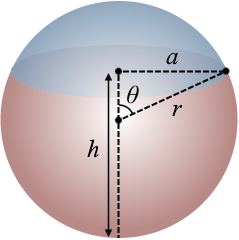# Sphere Volume Calculator

Created by Hanna Pamuła, PhD candidate
Reviewed by Bogna Szyk
Last updated: Apr 19, 2022

If you ever wondered what's the volume of the Earth, soccer ball or a helium balloon, our sphere volume calculator is here for you. It can help to calculate the volume of the sphere, given the radius or the circumference. Also, thanks to this calculator you can determine the spherical cap volume or hemisphere volume.

## Volume of a sphere formula

A sphere is a perfectly round geometrical 3D object. The formula for its volume equals:

volume = (4/3) * π * r³

Usually, you don't know the radius - but you can measure the circumference of the sphere instead, e.g., using the string or rope. The sphere circumference is the one-dimensional distance around the sphere at its widest point.

circumference = 2 * π * r, so r = circumference / (2 * π)

## How to find the volume of a sphere?

Do you know what the volume of an official FIFA World Cup soccer ball called size 5 is? Or basketball, size 7? Let's check!

1. Enter the radius of the sphere. For size 5 soccer ball radius should be equal to 4.3-4.5 in, let's take 4.4 in.
2. The sphere volume appeared, as the circumference. They are equal to 357 cu in and 27.6 in.
3. Assume that for the basketball we don't know the radius. Type in the circumference instead. For basketball size 7 the typical one is 29.5 in.
4. The volume of a sphere and radius is displayed, 433.5 cu in and 4.7 in, respectively.

Now try to calculate something else, take something bigger... Maybe do you want to know the volume of the Earth? The mean radius is approximately 6.37 x 106 m. The volume is then:

volume = (4/3) x π x (6370000 m)³ = 1,082,696,932,430,002,306,149 m³

## Spherical cap volume calculation

The spherical cap, called also spherical dome, is a portion of a sphere cut off by a plane. The formula behind its volume is:volume = ((π * h²) / 3) * (3r - h)  or
volume = (1/6) * π * h * (3a² + h²), where the radius of the sphere is r, the height of the cap (the blue one) is h, and a is radius of the base of the capWe can also use these formulas to find the volume of the opposite dome (the orange one), as shown in the illustration. However, make sure to use the correct measurement for h, which should always be the height of the spherical cap or dome we're interested in finding.

One example of the spherical dome is the fish tank, let's calculate how much water do we need to fill it:

1. Find the height of the cap. For example 7 in.
2. Determine the radius of the base of the cap. That is also the same as the radius of the fish tank's opening. Let's say it's equal to 3.1305 in.
3. Enter these values into our calculator. Upon doing so, our calculator will display the spherical cap volume to be equal to 287.35 cu in, and its corresponding sphere radius to be equal to 4.2 in.
4. To calculate the volume of the full sphere, use the basic calculator. Enter the radius 4.2 in.
5. Now you know, that our example fish tank has the volume 287.35 cu in, in comparison to 310.3 cu in for full sphere volume with the same radius.

## Hemisphere volume calculation

How to calculate it? Just use the formula for the spherical cap volume with the parameters equal to each other: sphere radius = height of the cap = cap base radius. Also, you can divide the full sphere result by 2 .

## Want more?

The sphere volume calculator is only one of our terrific volume tools, check out the others:

Hanna Pamuła, PhD candidate
Sphere
in
Circumference
inVolume
cu in
Spherical cap / Hemisphere
Cap height (h)
in
in
inVolume
cu in
People also viewed…

### Absolute value equation

This absolute value equation calculator will help you to solve equations that involve the absolute value of a linear expression. It shows intermediate calculations and graphs the solutions!

### Helium balloons

Wondering how many helium balloons it would take to lift you up in the air? Try this helium balloons calculator! 🎈

### Percentage increase

Percentage increase calculator calculates the increase of one value to the next in terms of percent.

### Secretary problem (Valentine's day)

Use the dating theory calculator to enhance your chances of picking the best lifetime partner.